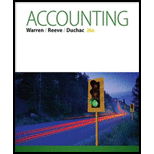Chapter 6, Problem 6.1EX### Accounting (Text Only)

26th Edition
Carl Warren + 2 others
ISBN: 9781285743615

#### Solutions

Chapter
Section### Accounting (Text Only)

26th Edition
Carl Warren + 2 others
ISBN: 9781285743615
Textbook Problem
21 views

# Determining gross profitDuring the current year, merchandise is sold for $4,885,000. The cost of the merchandise so ld is$3,028,700.a. What is the amount of the gross profit?b. Compute the gross profit percentage (gross profit divided by sales).c. Will the income statement necessarily report a net income? Explain.

a.

To determine

Gross profit represents the revenue after the deduction of cost of goods sold from the net sales of a business. Its mathematical representation is as below:

Gross profit=Net salesCost of goods sold

To Compute: The gross profit of Company.

Explanation

Calculate gross profit for Company.

Net sales = $4,885,000 Cost of goods sold =$3,028,700

Gross profit = Net sales Cost of goods sold=

b.

To determine

Gross profit percentage is the financial ratio that shows the relationship between the gross profit and net sales. Gross profit is the difference between the total revenues and cost of goods sold. It is calculated by using the following formula:

Gross profit percentage=Gross profitNet sales×100

To Compute: The gross profit percentage of the company.

c.

To determine

To Explain: Whether the income statement necessarily report a net income.

### Still sussing out bartleby?

Check out a sample textbook solution.

See a sample solution

#### The Solution to Your Study Problems

Bartleby provides explanations to thousands of textbook problems written by our experts, many with advanced degrees!

Get Started

#### Find more solutions based on key concepts